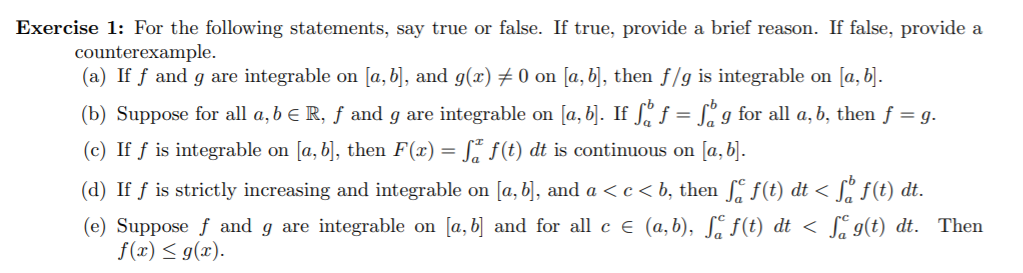# Exercise 1: For the following statements, say true or false. If true, provide a brief reason. If false, provide acounterexample.(a) If f and g are integrable on [a, b], and g(x) # 0 on [a, b], then f/g is integrable on [a, b].(b) Suppose for all a, b e R, ƒ and g are integrable on [a, b]. If Sº f = L° g for all a, b, then f = g.(c) If f is integrable on [a, b], then F(x) = S* f(t) dt is continuous on [a, b].(d) If ƒ is strictly increasing and integrable on [a, b], and a < c < b, then , f(t) dt < [, f(t) dt.(e) Suppose f and g are integrable on [a, b] and for all c e (a,b), S. f(t) dt < S g(t) dt. Thenf(x) < g(x).

Question
31 viewshelp_outlineImage TranscriptioncloseExercise 1: For the following statements, say true or false. If true, provide a brief reason. If false, provide a counterexample. (a) If f and g are integrable on [a, b], and g(x) # 0 on [a, b], then f/g is integrable on [a, b]. (b) Suppose for all a, b e R, ƒ and g are integrable on [a, b]. If Sº f = L° g for all a, b, then f = g. (c) If f is integrable on [a, b], then F(x) = S* f(t) dt is continuous on [a, b]. (d) If ƒ is strictly increasing and integrable on [a, b], and a < c < b, then , f(t) dt < [, f(t) dt. (e) Suppose f and g are integrable on [a, b] and for all c e (a,b), S. f(t) dt < S g(t) dt. Then f(x) < g(x). fullscreen
check_circle

star
star
star
star
star
1 Rating
Step 1

Hi! Your question is having multiple sub-parts. Since nothing is specified, we are answering only the first question. So, if you need the solution for other sub-parts then kindly post this question again with the specification of required sub-parts.

Step 2

We need to identify whether the statements are true or false with proper justifications.

Step 3

Now,

...

### Want to see the full answer?

See Solution

#### Want to see this answer and more?

Solutions are written by subject experts who are available 24/7. Questions are typically answered within 1 hour.*

See Solution
*Response times may vary by subject and question.
Tagged in
MathCalculus

### Integration